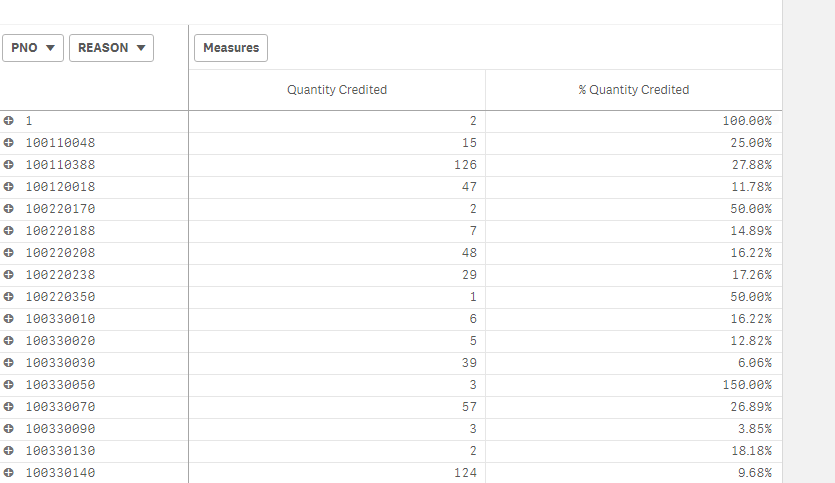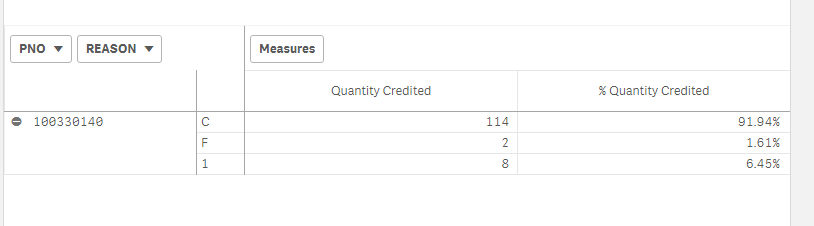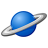Announcements
Sept. 27, 10AM ET Do more with Qlik, Insight Advisor – our intelligent AI-assistant in Qlik Sense: Register
cancel
Showing results for
Did you mean:Contributor

## How to show percentage of subtotal in pivot table?

I am trying to show percentage in pivot table in Qlik sense.Quantity credit is how many numbers of a particular PNO are returned. For example, out of total sales for PNO 100330140, the last one, 124 are returned for various reasons.

the percentage returned is 9.68%, that means 1281 parts were sold before taking credits into account.

The next level is reason which tells us out of 9.68% how many were returned for different reasons.This is what i wanted without selecting a part number, but on the pivot table itself. But when I expand pivot table for rows, I don't get desired results. I am getting percentage for whole data. Here I need 91.94%, 1.61%, and 6.45% for reasons C,F, and I respectively.

What I am getting is below :Formulae I am using for second measure (%Quantity Credited) is :

if(Dimensionality() <> 2,

fabs(Sum({<ORDTYPE={"CR"}>}TotalOrderQuantity))/sum({<ORDTYPE={"SL"}>}TotalOrderQuantity),

fabs(Sum({<ORDTYPE={"CR"}>}TotalOrderQuantity))/fabs(Sum(total{<ORDTYPE={"CR"}>}TotalOrderQuantity)))

Please let me know how to get desired result.

Thanks,

Vivek

1 Solution

Accepted SolutionsSpecialist

It should be

if(Dimensionality() <> 2,

fabs(Sum({<ORDTYPE={"CR"}>}TotalOrderQuantity))/sum({<ORDTYPE={"SL"}>}TotalOrderQuantity),

fabs(Sum({<ORDTYPE={"CR"}>}TotalOrderQuantity))/fabs(Sum(total <PNO> {<ORDTYPE={"CR"}}TotalOrderQuantity)))

4 RepliesSpecialist

You are using TOTAL which will ignore all dimensions, but you should ignore the first dimension:

if(Dimensionality() <> 2,

fabs(Sum({<ORDTYPE={"CR"}>}TotalOrderQuantity))/sum({<ORDTYPE={"SL"}>}TotalOrderQuantity),

fabs(Sum({<ORDTYPE={"CR"}>}TotalOrderQuantity))/fabs(Sum(total <first_Dim> {<ORDTYPE={"CR"}>}TotalOrderQuantity)))Contributor
Author

I used the following expression as well :

if(Dimensionality() <> 2,

fabs(Sum({<ORDTYPE={"CR"}>}TotalOrderQuantity))/sum({<ORDTYPE={"SL"}>}TotalOrderQuantity),

fabs(Sum({<ORDTYPE={"CR"}>}TotalOrderQuantity))/fabs(Sum(total PNO{<ORDTYPE={"CR"}}TotalOrderQuantity)))

It doesn't work.The thing is this work when I am selecting a part number.  the percentages are correct.  I believe there is an issue in aggregate because after selecting one part number, aggregate would be same.

Thanks,

VivekSpecialist

It should be

if(Dimensionality() <> 2,

fabs(Sum({<ORDTYPE={"CR"}>}TotalOrderQuantity))/sum({<ORDTYPE={"SL"}>}TotalOrderQuantity),

fabs(Sum({<ORDTYPE={"CR"}>}TotalOrderQuantity))/fabs(Sum(total <PNO> {<ORDTYPE={"CR"}}TotalOrderQuantity)))Contributor
Author

Thanks RakeshCommunity Browser